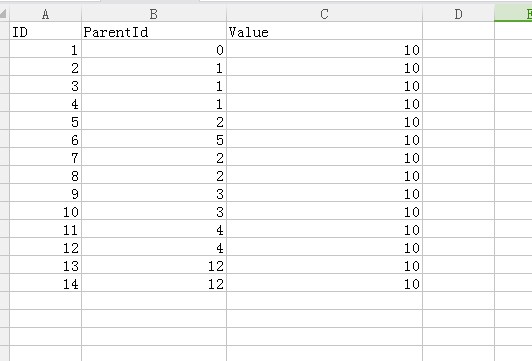•闪存
•博客
• 发言小组
• 投递新闻
• 提问博问
• 添加收藏
•文库

sql 树结构汇总直接下级，并去掉最大一个

0悬赏园豆：50 [待解决问题]2+5++6+7+8
3+10+11
4+11+12+13+14

0

public int fun(int id)
{

var sql = "select value from A where A.ParentId = "+ id;
List<A> list = context.A.execu(sql).List() //我随便写的，表示执行sql查询并返回一个list，查询所有属于1的子节点
int x =0;
foreach(A a in list)
{
//求子节点的value之和
x+=a.value;

//递归子树 x+=fun(a.ID); }
return x; }
int m =0;
public void fun2(int x)
{
//id为父节点，初始值为1
var sql = "select value from A where A.ParentId = "+ id;
List<A> list =context.A.execu(sql).List() //我随便写的，表示执行sql查询并返回一个list，查询所有属于1的子节点
foreach(A a in list)
{
m =max(m, fun(a.ID));
}
}
fun2(1);

Shendu.CC | 园豆：1918 (小虾三级) | 2018-03-19 22:50
0

0

mysql的话是不一样的，需要存储过程去写的

您需要登录以后才能回答，未注册用户请先注册